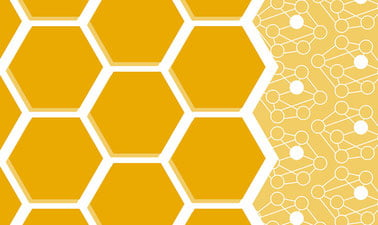# Data Structures & Algorithms IV: Pattern Matching, Dijkstra’s, MST, and Dynamic Programming Algorithms

Delve into Pattern Matching algorithms from KMP to Rabin-Karp. Tackle essential algorithms that traverse the graph data structure like Dijkstra’s Shortest Path. Study algorithms that construct a Minimum Spanning Tree (MST) from a graph. Explore Dynamic Programming algorithms. Use the course visualization tool to understand the algorithms and their performance.## There is one session available:

After a course session ends, it will be archived.
Estimated 5 weeks
9–10 hours per week
Self-paced

This Data Structures & Algorithms course completes the 4-course sequence of the program with graph algorithms, dynamic programming and pattern matching solutions. A short Java review is presented on topics relevant to new data structures covered in this course. The course does require prior knowledge of Java, object-oriented programming and linear and non-linear data structures. Time complexity is threaded throughout the course within all the data structures and algorithms.

You will delve into the Graph ADT and all of its auxiliary data structures that are used to represent graphs. Understanding these representations is key to developing algorithms that traverse the entire graph. Two traversal algorithms are studied: Depth-First Search and Breadth-First Search. Once a graph is traversed then it follows that you want to find the shortest path from a single vertex to all other vertices. Dijkstra’s algorithm allows you to have a deeper understanding of the Graph ADT. You will investigate the Minimum Spanning Tree (MST) problem. Two important, greedy algorithms create an MST: Prim’s and Kruskal’s.

Prim’s focuses on connected graphs and uses the concept of growing a cloud of vertices. Kruskal’s approaches the MST differently and creates clusters of vertices that then form a forest.

The other half of this course examines text processing algorithms. Pattern Matching algorithms are crucial in everyday technology. You begin with the simplest of the algorithms, Brute Force, which is the easiest to implement and understand. Boyer-Moore and Knuth-Morris-Pratt (KMP) improve efficiency by using preprocessing techniques to find the pattern. However, KMP does an exceptional job of not repeating comparisons once the pattern is shifted. The last pattern matching algorithm is Rabin-Karp which is an “out of the box” approach to the problem. Rabin-Karp uses hash codes and a “rolling hash” to find the pattern in the text. A different text processing problem is locating DNA subsequences which leads us directly to Dynamic Programming techniques. You will break down large problems into simple subproblems that may overlap, but can be solved. Longest Common Subsequence is such an algorithm that locates the subsequence through dynamic programming techniques.

### At a glance

• Institution: GTx
• Subject: Computer Science
• Level: Intermediate
• Prerequisites:

Basic knowledge of the Java programming language, object-oriented principles, and various abstract data types from LinkedLists to Hashmaps to Trees to Stacks & Queues.

# What you'll learn

Skip What you'll learn
• Improve Java programming skills by implementing graph and Dynamic Programming algorithms
• Study algorithm techniques for finding patterns in text processing
• Use preprocessing in the Boyer-Moore and KMP algorithms
• Explore the problem with hash codes in the Rabin-Karp algorithm
• Understand the Graph ADT and its representations within auxiliary structures
• Traverse graphs using the Depth-First and Breadth-First Search algorithms
• Investigate Dijkstra’s Shortest Path algorithm which operates on weighted graphs
• Study the Minimum Spanning Tree (MST) problem and its characteristics
• Utilize Greedy algorithms, like Prim’s and Kruskal’s, to find the MST
• Decompose large problems using Dynamic Programming techniques
• Apply Dynamic Programming techniques in the Longest Common Subsequence algorithm

# Syllabus

Skip Syllabus

Module 0: Introduction and Review

• Review of important Java principles involved in object-oriented design
• The Iterator & Iterable design patterns, and the Comparable & Comparator interfaces
• Basic “Big-Oh” notation and asymptotic analysis

Module 12: Pattern Matching Algorithms

• Examine algorithms for text processing, the simplest being Brute Force
• Apply preprocessing techniques in Boyer-Moore to improve performance
• Knuth-Morris-Pratt (KMP) avoids waste in prefixes of the pattern to achieve the best runtime
• Approach the pattern matching problem from the perspective of hash codes in Rabin-Karp
• Consider the time complexity of each of the algorithms

Module 13: Introduction to Graph Algorithms

• Explore the Graph ADT and its representation in auxiliary data structures
• Implement the Depth-First Search and Breadth-First Search graph traversal algorithms
• Examine weighted graphs and Dijkstra’s shortest path algorithm which uses edge relaxation

Module 14: Minimum Spanning Trees

• Study weighted, undirected graphs to find Minimum Spanning Trees (MST)
• Apply greedy algorithms to solve the MST problem
• Prim’s algorithm operates on connected graphs and employs the concept of cloud
• Approach the MST problem with Kruskal’s algorithm using cluster and forest concepts

Module 15: Dynamic Programming

• Apply the Dynamic Programming techniques that focus on the subproblems
• Examine the components of a dynamic programming algorithmic solution
• Implement the Longest Common Subsequence algorithm to solve DNA